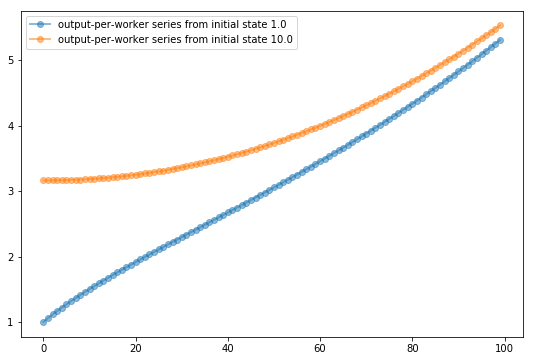# Basic Solow Growth Model (Solow_delong Python Class) ¶

Last edited: 2019-08-17

## The Pieces of the Model ¶

### Overview ¶

As is the case with all economic models, the Basic Solow Growth Model consists of:

• Variables: economic quantities of interest that we can calculate and measure, denoted by letters (or maybe two letters) like L for the number of workers in the labor force or Y for the amount of useful goods and services produced in a year—real GDP.

• Behavioral relationships: relationships that (1) describe how humans, making economic decisions given their opportunities and opportunity costs, decide what to do, and (2) that thus determine the values of the economic variables, represented by equations that have an economic variable on the left hand side and, on the right, a set of factors that determine the value of the variable and a rule of thumb for what that value is currently.

• Parameters: determine which out of a broad family of potential behavioral relations describes the behavior of the particular economic scenario at hand; the ability to work algebraically with parameters allows one to perform an enormous number of potential "what-if?" calculations very quickly and in a very small space
• Equilibrium conditions: conditions that tell us when the economy is in a position of balance, when some subset of the variables are "stable"—that is, are either constant are changing in simple and predictable ways, usually represented by solutions of some system of the equations that are behavioral relationships.

• Accounting identities: statements about the relationships between variables that are automatically and necessarily true because of the way the variables are defined, represented by equations.

In the case of the Solow growth model, the key variable is the capital intensity of the economy, which we will write using a lower-case Greek letter kappa: $\kappa$. We measure $\kappa$ as the ratio of the capital stock of the economy $K$, to the level of output and income $Y$.

In every economic model economists proceed with their analysis by looking for an equilibrium: a point of balance, a condition of rest, a state of the system toward which the model will converge over time. Economists look for equilibrium for a simple reason: either an economy is at its (or one of its) equilibrium position(s), or it is moving—and probably (or hopefully?) moving rapidly—to an equilibrium position. The Solow growth model is no exception.

Once economists have found the equilibrium position toward which the economy tends to move, they then understand how the model will behave. And, if they have built the right model, it will tell you in broad strokes how the economy will behave.

In the Basic Solow Growth Model, the equilibrium economists look for is an equilibrium in which the economy’s capital-output ratio $\kappa$ is contant, and thus the capital stock per worker, the level of income and output per worker, and the efficiency of labor are all three growing at exactly the same proportional rate—a rate that remains constant over time. We will call this rate g, for growth.

### The Production Function ¶

The first behavioral relationship in the Basic Solow Growth Model is the production function: the relationship between the economy's level of output and income $Y$ and its three derminants: the labor force $L$, the efficiency of labor $E$, and—depending on how we write it—either the economy's capital stock $K$ or its capital-output ratio $\kappa$. We want this behavioral relationship to satisfy three rules of thumb:

1. A proportional increase in the capital intensity of the economy will carry with it the same (smaller) proportional increase in income and production no matter how rich and productive the economy is. A 1% increase in capital intensity will always increase income and production by the same proportional amount.

2. If two economies have the same capital intensity, defined as the same capital-output ratio $\kappa$, and have the same level of technology- and organization-driven efficiency-of-labor $E$, then the ratio of their levels of income and output will be equal to the ratio of their labor forces $L$.

3. If two economies have the same capital intensity, defined as the same capital-output ratio $\kappa$, and have the same labor forces, then the ratio of their levels of income and output will be equal to the ratio of their technology- and organization-driven efficiencies-of-labor $E$.

There is one and only one way to write an algebraic expression that satisfies these two rule-of-thumb conditions. It is:

(1) $Y = \kappa^\theta E L$

You might object that this is circular: output and income $Y$ depend on capital intensity $\kappa$ defined as the capital-output ratio $K/Y$, but how can you calculate $\kappa$ and thus calculate $Y$ when you need to know $Y$ to calculate $\kappa$? If this worries you, you could start elsewhere, with a different-lookinig production function:

(2) $Y = K^\alpha (E L)^{1-\alpha}$

Then divide both sides by $Y^\alpha$:

(3) $\frac{Y}{Y^\alpha} = \frac{K^\alpha}{Y^\alpha} (E L)^{1-\alpha}$

(4) $Y^{1-\alpha} = \left(\frac{K}{Y}\right)^\alpha (E L)^{1-\alpha}$

(5) $Y^{1-\alpha} = \kappa^\alpha (E L)^{1-\alpha}$

(6) $Y^{\left(\frac{1-\alpha}{1-\alpha}\right)} = \kappa^{\left(\frac{\alpha}{1-\alpha}\right)} (E L)^{\left(\frac{1-\alpha}{1-\alpha}\right)}$

(7) $Y = \kappa^{\left(\frac{\alpha}{1-\alpha}\right)} E L$

which is exactly the same as (1), once we define $\alpha = \theta/(1+\theta)$ and thus $\theta = \alpha/(1-\alpha)$. It will often be convenient to work with $\alpha$. It will sometimes be convenient to work with $\theta$. It depends on which produces simpler formulas at any moment.

Why did Robert Solow back in 1956 look for an algebraic formula for his production function that would satisfy these three rules-of-thumb? Economists like to simplify, ruthlessly, when they can get away with it. Rule-of-thumb (1) is a simplifying assumption: an intellectual bet that the process of aggregate economic growth is likely to look very similar as an economy goes from an income-per-capita level of 10,000 to 20,000 dollars per worker per year as when it goes from 40,000 to 80,000 dollars per worker per year. It is worth making only as long as that is in fact true—that the similarities in the aggregate overall economic growth process in different decades and at different income-per-worker levels outweigh the differences. If that were not or were to cease being the case, we should drop that rule-of-thumb assumption. So far, so good.

Rule-of-thumb (2) is simply that holding other things—capital intensity and efficiency-of-labor—constant, you can always duplicate what you are doing and produce and earn twice as much. Again, it is worth making only as long as that is in fact true, or approximately true. Again, so far, so good.

Rule-of-thumb (3) is a recognition that better-organized economies making better use of technology will be more productive: it is best thought of as a definition of how we are going to construct our quantitative index of the level of applied technological knowledge combined with efficient economic organization—the variable $E$ that we call the efficiency-of-labor. It has no deeper implications.

### Savings, Investment, and Capital Accumulation¶

Following economists' custom of ruthless simplification, assume that individuals, households, and businesses desire to save a fraction $s$ of their income $Y$, so that total savings are:

(8) $S = sY$

We call s the economy’s saving rate or, more completely, its saving-investment rate (to remind us that s is measuring both the flow of saving into the economy’s financial markets and also the share of total production that is invested and used to build up and increase the economy’s capital stock).

Assume that there are no problems in translating individuals', households', and businesses' desires to save some of their income $Y$ into investment I:

(9) $I = S = sY$

While the saving-investment rate s is constant, the economy's capital stock K is not. It changes from year to year. Assume that there are no problems in translating investment $I$ into increases in the economy's productive stock $K$ of capital goods—and also assume that each year a fraction $\delta$ of the existing capital stock depreciates and wears out, so that the rate of change of the capital stock is:

(10) $\frac{dK}{dt} = sY - \delta K$

We typiclly assume that s is constant. We do, however, think about the consequences of its taking a permanent upward or downward jump at some particular moment of time. The background assumption, however—made because it makes formulas much simpler—will always be that s will then remain at its jumped-to value as far as we look into the future.

### Adding in Labor-Force and Population Growth¶

If the labor force L were constant and technological and organizational progress plus education that add to the efficiency-of-labor E were non-existent, we could immediately move on. But the economy’s labor force grows as more people turn 18 or so and join the labor force than retire, and as immigrants continue to arrive. And the efficiency of labor rises as science and technology progress, people keep thinking of new and more efficient forms of business organization, and people go to school and learn on the job

We assume—once again making a simplifying leap—that the economy’s labor force L is growing at a constant proportional rate n every year. Note that n is not the same across countries. Note that it can and does shift over time in any one country. But we want to tackle simple cases first. A constant labor force growth rate n is simple. Thus our background assumption will be that n is constant as far as we can see into the future. Then between this year and the next the labor force grows according to the formula:

(11) $\frac{dL_t}{dt} = nL_t$

Next year’s labor force will thus be n percent higher than this year’s labor force.

### Efficiency-of-Labor Growth¶

We also assume—once again making a simplifying leap—that the economy’s efficiency of labor E is growing at a constant proportional rate g every year. Note that g is not the same across countries. Note that it can and does shift over time in any one country. But we want to tackle simple cases first. A constant efficiency-of-labor growth rate g is simple. Thus our background assumption will be that g is constant as far as we can see into the future. Then between this year and the next the efficiency of labor grows according to the formula:

(12) $\frac{dE_t}{dt} = gE_t$

### Definition of Capital-Output Ratio $\kappa$¶

And we define the economy's capital intensity $\kappa$ as:

(13) $κ_t = \frac{K_t}{Y_t}$

the economy's capital-output ratio

## Solving for Equilibrium ¶

### Calculating the Rate-of-Change of Capital Intensity $\kappa$ ¶

Begin with our alternative equation for output:

(14) $Y = K^\alpha (L E)^{1-alpha}$

Take natural logarithms:

(15) $\ln(Y) = \alpha\ln(K) + (1-\alpha)(\ln(L) + \ln(E)$

Take the time derivative of both sides, and remember that the proportional growth rates of L and E are n and g, respectively:

(16) $\frac{1}{Y}\frac{dY}{dt} = α \left( \frac{1}{K}\frac{dK}{dt} \right) + (1-α)(n+g)$

Likewise take the time derivative of the capital stock, and divide both sides by the level of the capital stock:

(17) $\frac{1}{K}\frac{dK}{dt} = \frac{s}{κ} - δ$

Recognize that the proportional rate of growth of the economy's capital intensity is simply the difference between the proportional growth rates of the capital stock K and of output and income Y:

(18) $\frac{1}{κ}\frac{dκ}{dt} = \frac{1}{K}\frac{dK}{dt} - \frac{1}{Y}\frac{dY}{dt}$

(19) $\frac{1}{κ}\frac{dκ}{dt} = \frac{1}{K}\frac{dK}{dt} - α \left( \frac{1}{K}\frac{dK}{dt} \right) - (1-α)(n+g)$

(20) $\frac{1}{κ}\frac{dκ}{dt} = (1- α) \left( \frac{1}{K}\frac{dK}{dt} \right) - (1-α)(n+g)$

(21) $\frac{1}{κ}\frac{dκ}{dt} = (1- α) \left( \frac{s}{κ} - δ \right) - (1-α)(n+g)$

(22) $\frac{dκ}{dt} = (1- α) \left( s - (n+g+δ)κ \right)$

### Exponential Convergence ¶

Let's add a little time subscript to recognize that the capital intensity $\kappa$ of the economy varies over time:

Integrating:

(23) $\frac{dκ_t}{dt} = (1- α) \left( s - (n+g+δ)κ_t \right)$

And let us suppose that we know that at some start time—call it $t = 0$ the value of $\kappa$ is some known quantity, which we will call $\kappa_0$. Then we can integrate forward from $t = 0$ and get the value of the economy's capital intensity $\kappa$ as far into the future as we wish:

(24) $κ_t = \frac{s}{n+g+δ} + e^{-(1-α)(n+g+δ)t} \left[ κ_0 - \frac{s}{n+g+δ} \right]$

Note that as time passes, $\kappa_t$ exhibits exponential convergence towards an asymptotic steady-state value $\kappa^*$:

(25) $\kappa^* = \frac{s}{n +g + \delta}$

And note that the exponential convergence rate $r_{c\kappa}$ is:

(26) $r_{c\kappa} = (1-\alpha)(s +g + \delta)$

### Other Initial Parameter Values ¶

It is not enough to just know the values of the behavioral-relationship parameters $s, \alpha, n, g,$ and $\delta$ and the value at time $t = 0$ of the capital-intensity $\kappa_0$. We also need to know the initial values of the labor force $L_0$ and the efficiency-of-labor $E_0$. But if we know all of these we can then calculate the evolution of the economy according to the Basic Solow Growth model. The economic variables will be:

The labor force:

(30) $L_t = L_0 e^{nt}$

The efficiency-of-labor:

(31) $E_t = E_0 e^{gt}$

Total income and output:

(32) $Y_t = (\kappa)^\theta (L E) = (\kappa)^{\alpha/(1-\alpha)} (L E)$

The capital stock:

(33) $K_t = \kappa Y_t$

And income per worker:

(34) $y_t = \frac{Y_t}{L_t} = (\kappa)^\theta E) = (\kappa)^{\alpha/(1-\alpha)} (L E)$

## Simulations ¶

### Calculus and Algebra, or Arithmetic and Python? ¶

We could use the calculus and algebra above to answer questions about how an economy well-modeled by the Basic Solow Growth Model behaves. Or we could take a different tack, and simply use arithmetic and Python to answer such questions by simulating an economy that behaves according to that model.

### Moving to a Discrete Time Model ¶

We cannot perform simulations in continuous time: we have to let the time in our model simulations tick forward step by step. We have to discretize. Discretizing for a difference model:

(27) $κ_{t+1} = κ_t + \left[ \frac{1 - e^{-(1-α)(n+g+δ)}}{n+g+δ} \right] \left( s - (n+g+δ)κ_t \right)$

(28) $1 - α' = (1 - α) [ 1 - \frac{(1-α)(n+g+δ)}{2} + \frac{(1-α)^2(n+g+δ)^2}{6} - ... ]$

(29) $κ_{t+1} = κ_t + (1- α') \left( s - (n+g+δ)κ_t \right)$

Almost always we will ignore the difference between the continuous-time $\alpha$ and the discrete-time $\alpha'$: the difference will almost always be too small to matter (much).

### The Solow_delong Python Class ¶

Immediately below is a code cell containing the definition of the Solow_delong Python class. It takes initial parameter values for $\alpha, s, n, g, \delta, E_t, L_t$, and $\kappa_t$ at some moment t, and calculates th4 values of all the relevant economic variables in the following year t+1. It can then save the updated values and call itself in to construct and report the time series values for an economy following the Basic Solow Growth Model for some number T of periods into the future:

Two cells below is a code cell that uses the Solow_delong Python class to construct a six-panel figure graphing time series for the most interesting economic variables in the Basic Solow Growth Model for T periods into the future from an arbitrary starting point. The graphs show what happens both according to a baseline scenario, and according to an an alternative scenario showing what happened after parameters did or what would have happened had parameters jumped:

In :
# DEFINING THE delong_classes.solow PYTHON CLASS
#
# kept in: delong_classes.py

import numpy as np
import matplotlib.pyplot as plt
%matplotlib inline

class solow:

"""
Implements the Solow growth model calculation of the
capital-output ratio κ and other model variables
using the update rule:

κ_{t+1} = κ_t + ( 1 - α) ( s - (n+g+δ)κ_t )

Built upon and modified from Stachurski-Sargeant
<https://quantecon.org> class **Solow**
<https://lectures.quantecon.org/py/python_oop.html>
"""

def __init__(self, n=0.01,              # population growth rate
s=0.20,              # savings rate
δ=0.03,              # depreciation rate
α=1/3,               # share of capital
g=0.01,              # productivity
κ=0.2/(.01+.01+.03), # current capital-labor ratio
E=1.0,               # current efficiency of labor
L=1.0):              # current labor force

self.n, self.s, self.δ, self.α, self.g = n, s, δ, α, g
self.κ, self.E, self.L = κ, E, L
self.Y = self.κ**(self.α/(1-self.α))*self.E*self.L
self.K = self.κ * self.Y
self.y = self.Y/self.L
self.α1 = 1-((1-np.exp((self.α-1)*(self.n+self.g+self.δ)))/(self.n+self.g+self.δ))
self.initdata = vars(self).copy()

def calc_next_period_kappa(self):
"Calculate the next period capital-output ratio."
# Unpack parameters (get rid of self to simplify notation)
n, s, δ, α1, g, κ= self.n, self.s, self.δ, self.α1, self.g, self.κ
# Apply the update rule
return (κ + (1 - α1)*( s - (n+g+δ)*κ ))

def calc_next_period_E(self):
"Calculate the next period efficiency of labor."
# Unpack parameters (get rid of self to simplify notation)
E, g = self.E, self.g
# Apply the update rule
return (E * np.exp(g))

def calc_next_period_L(self):
"Calculate the next period labor force."
# Unpack parameters (get rid of self to simplify notation)
n, L = self.n, self.L
# Apply the update rule
return (L*np.exp(n))

def update(self):
"Update the current state."
self.κ =  self.calc_next_period_kappa()
self.E =  self.calc_next_period_E()
self.L =  self.calc_next_period_L()
self.Y = self.κ**(self.α/(1-self.α))*self.E*self.L
self.K = self.κ * self.Y
self.y = self.Y/self.L

"Compute the steady state value of the capital-output ratio."
# Unpack parameters (get rid of self to simplify notation)
n, s, δ, g = self.n, self.s, self.δ, self.g
# Compute and return steady state
return (s /(n + g + δ))

def generate_sequence(self, T, var = 'κ', init = True):
"Generate and return time series of selected variable. Variable is κ by default. Start from t=0 by default."
path = []

# initialize data
if init == True:
for para in self.initdata:
setattr(self, para, self.initdata[para])

for i in range(T):
path.append(vars(self)[var])
self.update()
return path

In :
# SIX-PANEL BASIC SOLOW GROWTH MODEL FIGURE
#
# Here we graph time series for the six most interesting
# economic variables in the Basic Solow Growth Model
# for T periods into the future from an arbitrary starting
# point. We graph both a baseline scenario, and an
# alternative scenario showing what happened alternatively
# after a jump in parameters, or what would have happened

import numpy as np
import matplotlib.pyplot as plt
%matplotlib inline

import delong_classes

T = 100

s_base = delong_classes.solow(κ=4.0)
s_base.scenario = "base scenario"
s_alt = delong_classes.solow(κ=0.5)
s_alt.scenario = "alt scenario"

figcontents = {
(0,0):('κ','Capital Output'),
(0,1):('E','Efficiency of Labor'),
(1,0):('L','Labor Force'),
(1,1):('K','Capital Stock'),
(2,0):('Y','Output'),
(2,1):('y','Output-per-worker')
}

num_rows, num_cols = 3,2
fig, axes = plt.subplots(num_rows, num_cols, figsize=(12, 12))
for i in range(num_rows):
for j in range(num_cols):
for s in s_base, s_alt:
lb = f'{s.scenario}: initial κ = {s.initdata["κ"]}'
axes[i,j].plot(s.generate_sequence(T, var = figcontents[i,j]),'o-', lw=2, alpha=0.5, label=lb)
axes[i,j].set(title=figcontents[i,j])

#   global legend
axes[(0,0)].legend(loc='upper center', bbox_to_anchor=(1.1,1.3))
plt.suptitle('Solow Growth Model: Simulation Run', size = 20)
plt.show()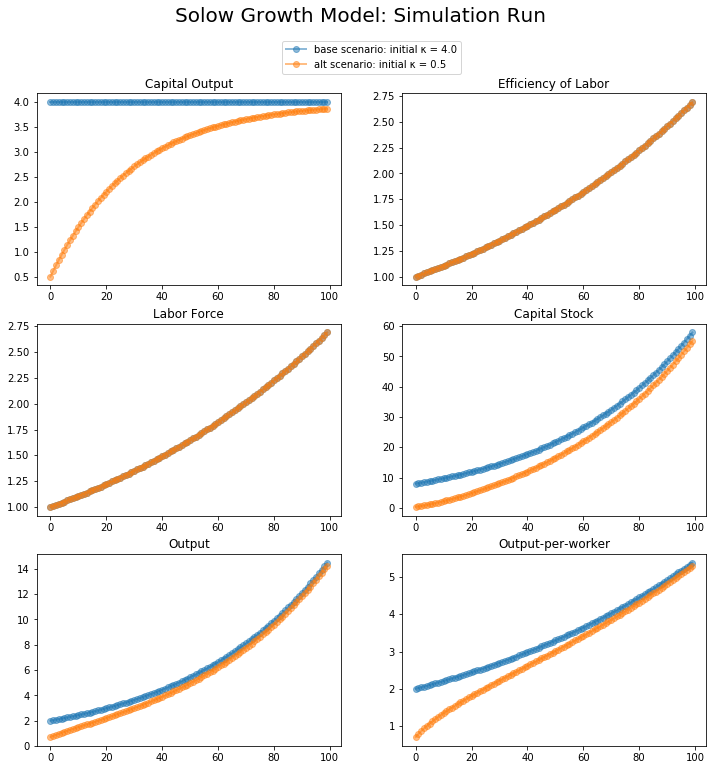In :
# ONE PANEL CAPITAL-OUTPUT RATIO FIGURE
#
# Plotting the economy's capital intensity
# for a _base_line and an _alt_ernative
# scenario

import numpy as np
import matplotlib.pyplot as plt
%matplotlib inline

import delong_classes

T = 250

Economy = delong_classes.solow(κ=1.0, α=0.25)

fig, ax = plt.subplots(figsize=(9, 6))

# Plot the steady-state value of the capital-output ratio

# Plot the capital-output ratio over time

lb = f'α parameter value {Economy.initdata["α"]}'
ax.plot(Economy.generate_sequence(T), 'o-', lw=2, alpha=0.5, label=lb)

ax.legend()
plt.show()

Economy = delong_classes.solow(κ=1.0, α=0.5)

fig, ax = plt.subplots(figsize=(9, 6))

# Plot the steady-state value of the capital-output ratio

# Plot the capital-output ratio over time

lb = f'α parameter value {Economy.initdata["α"]}'
ax.plot(Economy.generate_sequence(T), 'o-', lw=2, alpha=0.5, label=lb)

ax.legend()
plt.show()

Economy = delong_classes.solow(κ=1.0, α=0.75)

fig, ax = plt.subplots(figsize=(9, 6))

# Plot the steady-state value of the capital-output ratio

# Plot the capital-output ratio over time

lb = f'α parameter value {Economy.initdata["α"]}'
ax.plot(Economy.generate_sequence(T), 'o-', lw=2, alpha=0.5, label=lb)

ax.legend()
plt.show()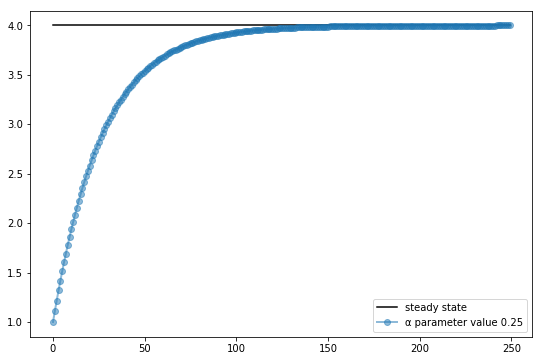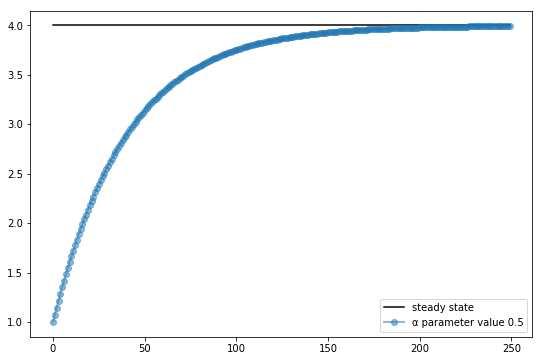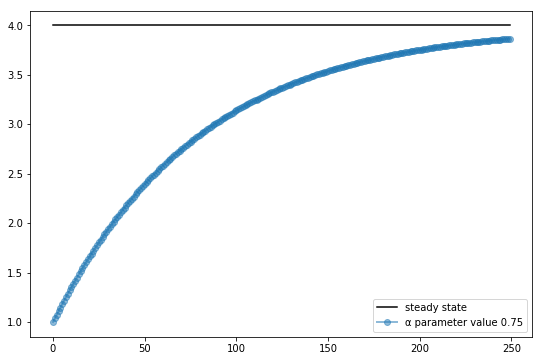In :
# SPEED OF CONVERGENCE FOR LOW, MEDIUM, AND HIGH α

import numpy as np
import matplotlib.pyplot as plt
%matplotlib inline

import delong_classes

T = 100

s_base = delong_classes.solow(κ=1.0, )
s_alt = delong_classes.solow(κ=10.0)

fig, ax = plt.subplots(figsize=(9, 6))

# Plot the common steady-state value of the capital-output ratio

# Plot time series for each economy
for s in s_base, s_alt:
lb = f'capital-output series from initial state {s.initdata["κ"]}'
ax.plot(s.generate_sequence(T), 'o-', lw=2, alpha=0.5, label=lb)

ax.legend()
plt.show()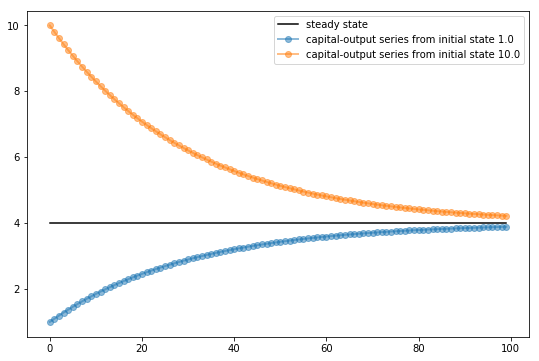## Basic Solow Growth Model (Solow_delong Python Class) ¶

### Capital Intensity $\kappa = K/Y$ as State Variable ¶### Change Log¶

2019-07-25 by DeLong: Playing with Sargent and Stachurowski's QuantEcon: https://quantecon.org

2019-08-05 by Yinshan, list of changes made:

1. Merged generate_x_sequence into one method
2. Added initializing steps to generate_sequence method to eliminate the need of redefining instance for every plot
3. Removed unnecessary import commands
4. Changed comment to docstring for the class SolowKappa
5. Changed $\alpha$ to $\alpha'$ in calculation of $\kappa$

Effect: slight change in $\kappa$ value for each time period (converge slower to the steady state), but no change in general convergence behavior

6. Modified unpacking commands for self variables, removed unused ones and added used ones

2019-08-07 by delong: list of changes made:

1. Named s_base and s_alt cases
2. Added the six-panel summary graph

2019-08-08 by delong: list of changes made:

1. Renamed SolowKappa class to Solow_delong

2019-08-08 by Yinshan: list of changes made:

1. Modified six-panel summary code, added loop and figcontents dictionary
2. Changed six-panel summary graph legend to global, added subtitles to subplots
self.initdata = vars(self).copy()
and  if init == True: for para in self.initdata:
  setattr(self, para, self.initdata[para])



2019-08-17 by DeLong: typos corrected:

2019-08-18 by DeLong: Added verbiage: model explication. Added equations: filling in some steps.

## Appendices¶

Illustration on selected syntaxes:

1. self.initdata = vars(self).copy()

vars(self) returns a dictionary containing data attributes of the instance self.

More technically speaking, for every module object in Python, the read-only attribute __dict__ returns the dictionary used to implement the module’s namespace.

In our case, vars(self) gives us all initial data attributes we just defined. self.initdata = vars(self).copy() copies the returned dictionary and stores it for future use in the initializing step of generate_sequence method.

2. if init == True:
for para in self.initdata:
setattr(self, para, self.initdata[para])

The above steps initialize all parameters stored in self.initdata. setattr is used to assigns a value to the attribute, provided the object allows it. Usually setattr(object,name,value) is equivalent to object.name = value.

However, in our case, we need to get attributes dynamically named in a loop, depending on current para. setattr gives a handy solution. The usual = way of assigning value would return an error.

In :
# change starting points
s_base = delong_classes.solow(κ=1.0)

s_alt = delong_classes.solow(κ=10.0)

# reproduce plots
num_rows, num_cols = 3,2
fig, axes = plt.subplots(num_rows, num_cols, figsize=(12, 12))
for s in s_base, s_alt:
for i in range(num_rows):
for j in range(num_cols):
lb = f'initial κ = {s.initdata["κ"]}'
axes[i,j].plot(s.generate_sequence(T, var = figcontents[i,j]),'o-', lw=2, alpha=0.5, label=lb)
axes[i,j].set(title=figcontents[i,j])

#   global legend
axes[(0,0)].legend(loc='upper center', bbox_to_anchor=(1.1,1.3),ncol=3)

plt.suptitle('Solow Growth Model: Simulation Run', size = 20)
plt.show()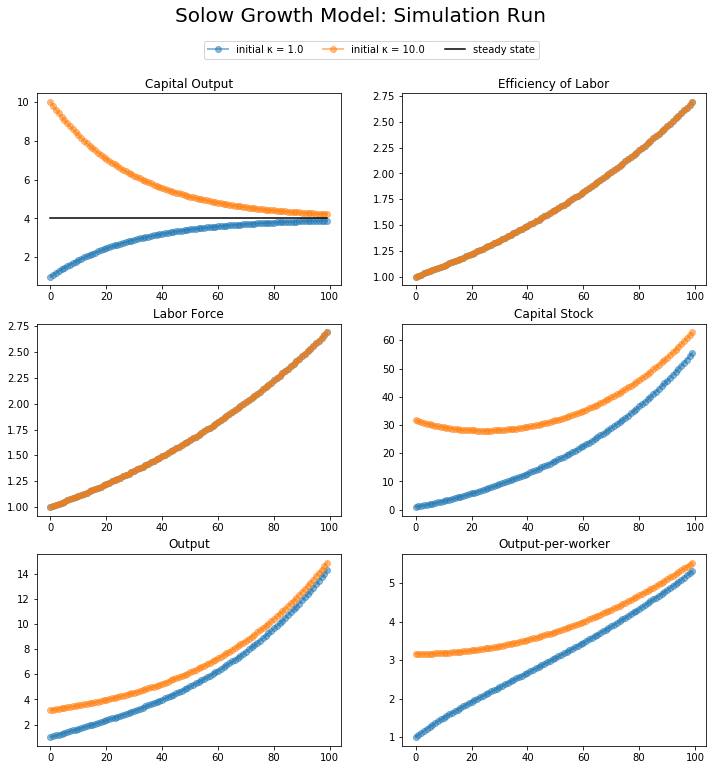In :
fig, ax = plt.subplots(figsize=(9, 6))

# Plot time series for each economy
for s in s_base, s_alt:
lb = f'efficiency-of-labor series from initial state {s.initdata["κ"]}'
ax.plot(s.generate_sequence(T, var='E'), 'o-', lw=2, alpha=0.5, label=lb)

ax.legend()
plt.show()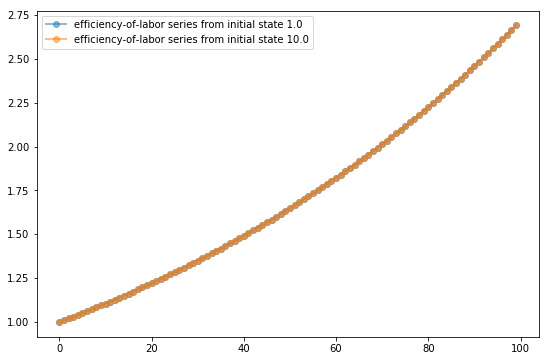In :
fig, ax = plt.subplots(figsize=(9, 6))

# Plot time series for each economy
for s in s_base, s_alt:
lb = f'labor-force series from initial state {s.initdata["κ"]}'
ax.plot(s.generate_sequence(T, var = 'L'), 'o-', lw=2, alpha=0.5, label=lb)

ax.legend()
plt.show()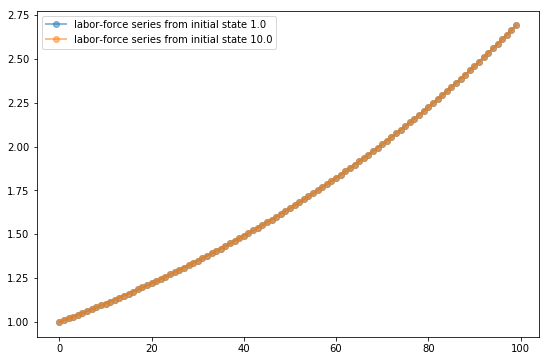In :
fig, ax = plt.subplots(figsize=(9, 6))

# Plot time series for each economy
for s in s_base, s_alt:
lb = f'capital-stock series from initial state {s.initdata["κ"]}'
ax.plot(s.generate_sequence(T, var = 'K'), 'o-', lw=2, alpha=0.5, label=lb)

ax.legend()
plt.show()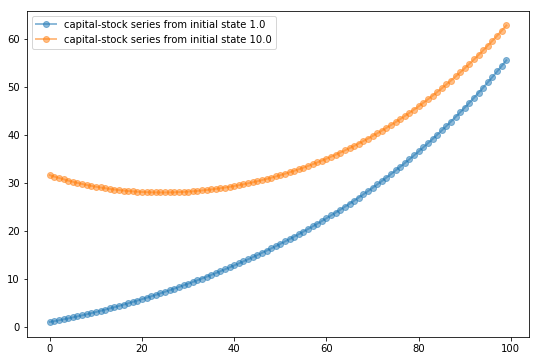In :
fig, ax = plt.subplots(figsize=(9, 6))

# Plot time series for each economy
for s in s_base, s_alt:
lb = f'output series from initial state {s.initdata["κ"]}'
ax.plot(s.generate_sequence(T, var = 'Y'), 'o-', lw=2, alpha=0.5, label=lb)

ax.legend()
plt.show()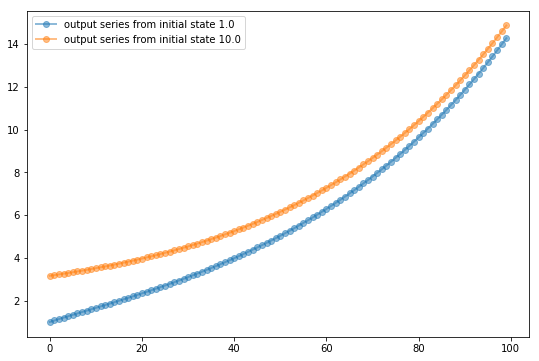In :
fig, ax = plt.subplots(figsize=(9, 6))

for s in s_base, s_alt:
lb = f'output-per-worker series from initial state {s.initdata["κ"]}'
ax.plot(s.generate_sequence(T, var = 'y'), 'o-', lw=2, alpha=0.5, label=lb)
ax.legend()

plt.show()